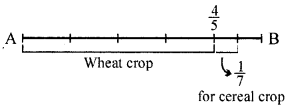## ML Aggarwal Class 6 Solutions for ICSE Maths Chapter 6 Fractions Check Your Progress

Question 1.
State whether the following statements are true (T) or false (F):
(i) The fraction $$\frac{2}{3}$$ lies between 2 and 3.
(ii) To find an equivalent fraction to a given fraction, we may add or subtract the same (non-zero) number to its numerator and denominator.
(iii) To add or subtract like fractions, we add or subtract the numerators while keeping the denominator same.
Solution:
(i) False
As $$\frac{2}{3}$$ lies between 0 and 1
(ii) False
We should multiply and divide by appropriate numerator keep the denominator same. And that appropriate number is obtained by the LCM of all the denominators.
(ii) True

Question 2.
How many natural numbers are there between 102 and 112? What fraction of them are prime numbers?
Solution:
The natural numbers from 102 to 112 are: 103, 104, 105, 106, 107, 108, 109, 110, 111 Total number of natural numbers = 9 Out of these prime numbers are:
103, 107, 109 = 3
∴ Total number of these prime numbers = 3
Required fraction = $$\frac{3}{9}=\frac{1}{3}$$

Question 3.
Match the equivalent fractions from each row: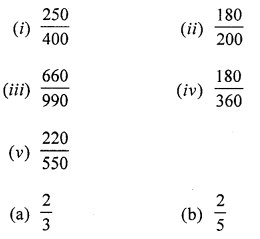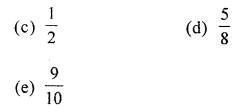Solution: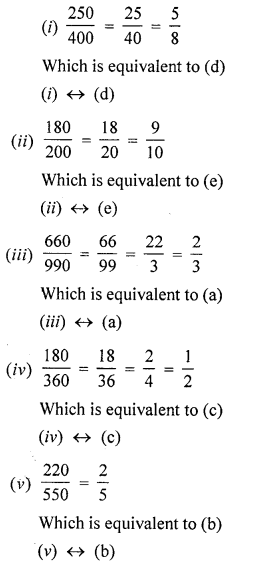Question 4.
Replace by an appropriate symbol ‘< or >’ between the given fractions:Solution:
(i) $$\frac{5}{6} \dots \frac{13}{15}$$
LCM of 6 and 15
= 3 × 2 × 5 = 30
Now, making them like fractions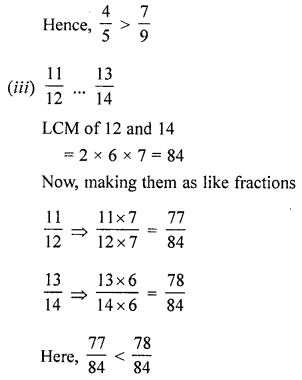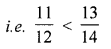Question 5.
Arrange the following fractions in descending order : $$\frac{7}{30}, \frac{13}{15}, \frac{9}{10}, \frac{3}{5}$$
Solution:Question 6.
Simplify: $$2 \frac{1}{2}-3 \frac{1}{4}+5 \frac{5}{6}$$
Solution: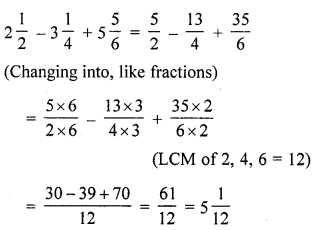Question 7.
Evaluate the following:Solution:Question 8.
Asha and Samuel have bookshelves of the same size partly filled with books. Asha’s shelf is $$\frac{5}{6}$$ full and Samuel’s shelf is $$\frac{3}{5}$$ full. Whose bookshelf is more full and by what fraction?
Solution:
Asha’s shelf is $$\frac{5}{6}$$th fukk of book Samuel’s shelf is $$\frac{3}{5}$$th full of book. [LCM (6, 5) = 30]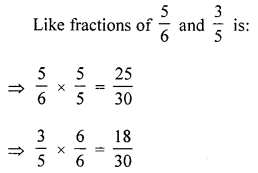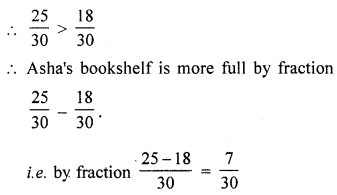Question 9.
A farmer uses four out of five equal strips of his land for wheat crop and $$\frac{1}{7}$$ of his land for cereal crop. What fraction of his land is available for other crops?
Solution:
A farmer has 5 equal strips of land